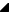﻿ TRSChartAxis.PixelToAxis(TCanvasPixel,TCanvasRect,Boolean) Method# TRSChartAxis.PixelToAxis(TCanvasPixel,TCanvasRect,Boolean) Method

Converts from a canvas pixel coordinate to a chart axis coordinate. Based on the    ZoomMinimum and    ZoomMaximum range of the chart axis, this routine converts the parameter value into a chart axis coordinate. This method uses the ChartRect parameter to specify the visual pixel range of the chart axis. The chart axis uses the bounds of the rectangle that apply to its location, e.g., a BottomAxis chart axis would use the ChartRect's Left=ZoomMinimum and Right=ZoomMaximum fields for the calculation (the rectangle is also adjusted if StartPosition and EndPosition properties are not their defaults).

Use one of the PixelToAxis methods anytime you need to convert from a canvas pixel coordinate to the internal chart axis coordinates. For example, if the chart axis has a zoomed range from 0 to 100 and the ChartRect is Left=150 and Right=500, this function would return:
PixelToAxis( 150 ) = 0
PixelToAxis( 325 ) = 50
PixelToAxis( 500 ) = 100

Use one of the AxisToPixel methods to convert back from chart coordinates to canvas pixel coordinates.

Namespace: RSChartPanelSyntax
 Delphi public    function PixelToAxis( Value: TCanvasPixel; const ChartRect: TCanvasRect; IgnoreZoom: Boolean = False ): TRSChartValueType; overload;

Parameters

Value

Type: TCanvasPixel

ChartRect

Type: TCanvasRect

IgnoreZoom

Type: Boolean

#### Return Value

Type: TRSChartValueTypeExamples
 The following code displays the chart axes' coordinates for the point under the mouse: Delphi procedure TForm1.RSChartPanel1MouseMove(Sender: TObject;    Shift: TShiftState; X, Y: Integer);  begin       with Sender as TRSChartPanel do            Self.Caption := 'X='+FloatToStr(BottomAxis.PixelToAxis(X)) + ' ' +                            'Y='+FloatToStr(LeftAxis.PixelToAxis(Y));  end;

The following code does the same thing for the paint box:

Delphi

procedure TForm1.PaintBox1MouseMove(Sender: TObject; Shift: TShiftState; X,
Y: Integer);
begin
with Sender as TPaintBox do
Self.Caption := 'X='+FloatToStr(RSChartPanel1.BottomAxis.PixelToAxis(X, Canvas.ClipRect)) + ' ' +
'Y='+FloatToStr(RSChartPanel1.LeftAxis.PixelToAxis(Y, Canvas.ClipRect));
end;See Also

RiverSoftAVG Charting Component Suite (RCCS) © 2005-2015, Thomas G. Grubb